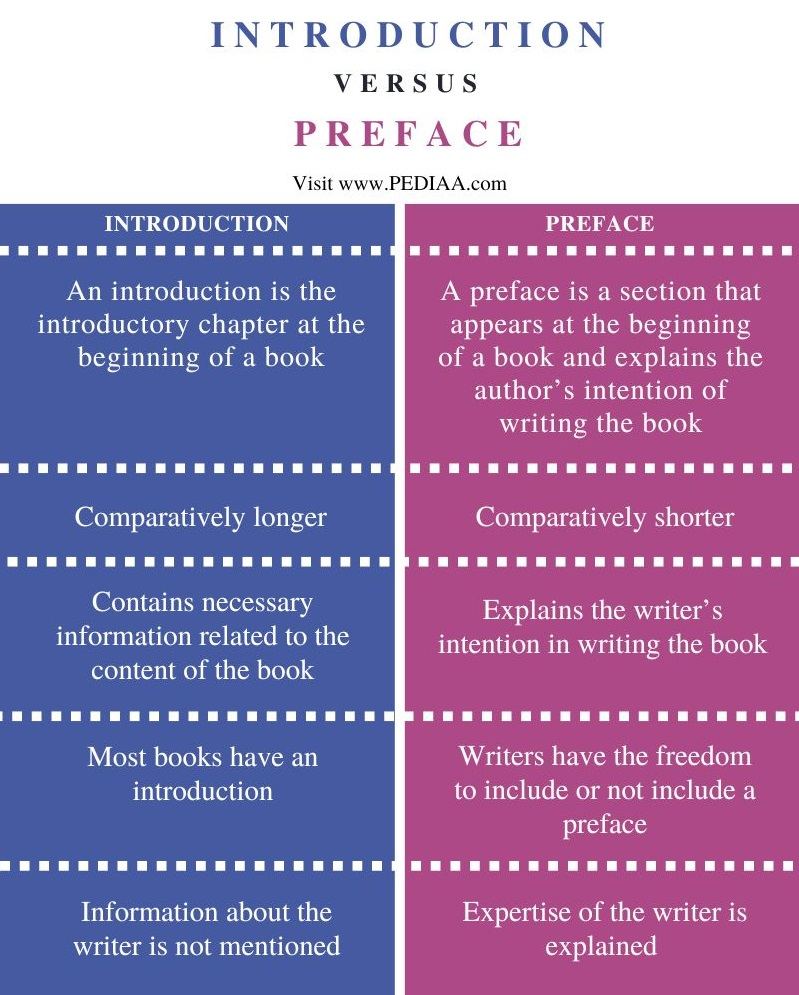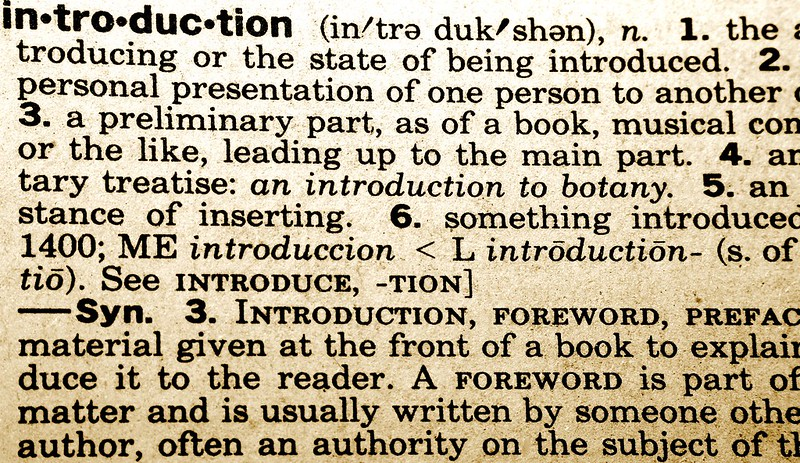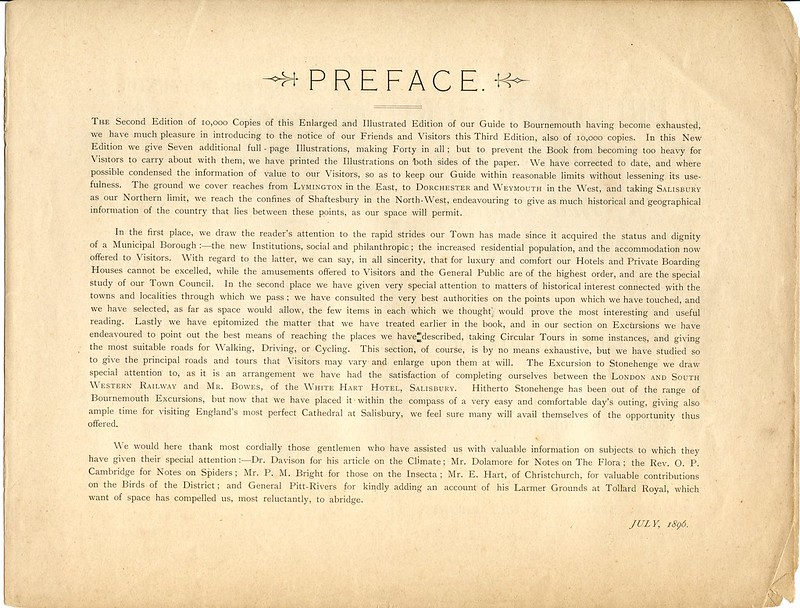# What is the Difference Between Introduction and Preface

The main difference between introduction and preface is that introduction of a book is much more connected to the content of the book, while preface allows the writer to introduce himself and explain about aims of his writing to the readers.

Both introduction and preface appear at the beginning of a book. This is why most readers assume them to be the same. However, there is a distinct difference between introduction and preface.

### Key Areas Covered

1. What is an Introduction
– Definition, Features
2. What is a Preface
– Definition, Features
3. Similarities Between Introduction and Preface
– Outline of Common Features
4. Difference Between Introduction and Preface
– Comparison of Key Differences

### Key Terms

Introduction, Preface## What is an Introduction

An introduction refers to the introductory chapter at the beginning of a book. The introduction includes basic and necessary information regarding the content of the book. Most of the time, an introduction offers facts relevant to the subject of the book. Thus, by reading the introduction, readers can get a better understanding of the content of the book.For non-fiction and fiction, the introduction chapter functions in different ways. In non-fiction, the author can include the definitions and concepts that are relevant for the rest of the chapters in the introductory chapter. On the other hand, in fiction, the introduction hints at the plot of the story and gives some signals about the story to the readers. There are no specific or standard lengths for an introduction. Writers have the freedom to include the information as necessary. Since an introduction chapter starts the content of the book, most writers treat the introduction chapter just like other chapters and take care to keep the length in line with the other chapter lengths. Another important fact related to the introduction is that the information included in the introductory chapter should have relevance to the reader. If the introductory chapter includes unnecessary information, it may reduce readers’ interest in the book.

## What is a Preface

A preface appears at the beginning of a book and explains the author’s intention in writing the book. A preface does not contain information about the relevant book. Prefaces are most common in non-fiction books, but there are instances where fictions also use a preface at the beginning of the book. Here, the writer gets a chance to state what has inspired him or her to write the book as well as to explain the importance of the book to the reader. This also becomes a platform for the writer to mention the credibility of his or her expertise in the relevant field.Using a preface, writers can arouse the curiosity of the readers about the content of the book. Furthermore, writers can directly talk about the purpose and importance of the book in a preface. However, writers should take care to raise readers’ interest in the book and subject using the preface rather than boosting their ego. The format of a preface generally includes the signature of the author as well as the date and time of writing. This is an effective way to indicate that the writer is writing hindsight.

## Similarities Between Introduction and Preface

• Both preface and introduction appear at the beginning of a book.
• Both fiction and nonfiction books can have prefaces and introductions.

## Difference Between Introduction and Preface

### Definition

An introduction refers to the introductory chapter at the beginning of a book, while a preface is a section that appears at the beginning of a book and explains the author’s intention of writing the book.

### Length

An introductory chapter is usually longer than a preface.

### Content

The content of a preface includes the writer’s intention in writing the book, whereas an introductory chapter contains necessary information related to the content of the book.

### Relevance

Although writers have the freedom to include or not include a preface to their book, an introductory chapter must be included in a book.

### Background Information

Although the expertise of the writer is explained in the preface, the information about the writer is not mentioned in the introductory chapter.

### Conclusion

Both the introduction and preface appear at the beginning of a book. The main difference between introduction and preface is that introduction of a book is much more connected to the content of the book, while the preface allows the writer to introduce himself and explain about aims of his writing to the readers.

##### Reference:

1. “What is a Preface?” Reedsy Blog.

##### Image Courtesy:

1. “Introduction” By Larah McElroy (CC BY-NC 2.0) via Flickr
2. “1896 Bright’s Guide preface” By Alwyn Ladell (CC BY-NC-ND 2.0) via Flickr